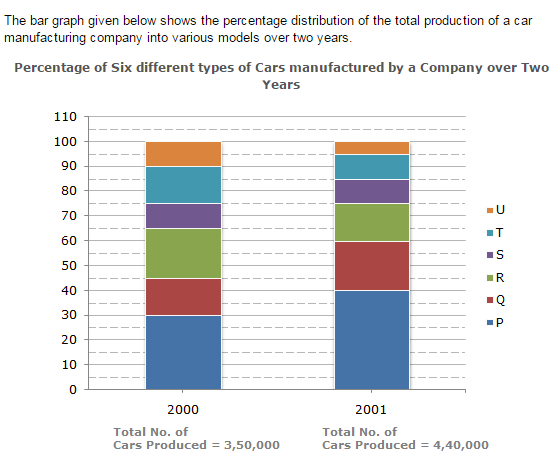Direction1.

What was the difference in the number of Q type cars produced in 2000 and that produced in 2001?

 A. 35,500 B. 27,000 C. 22,500 D. 17,500
Explanation: Total number of Q type cars produced in 2001 =(60 - 40)% of 4,40,000 = 88,000. Total number of Q type cars produced in 2000 =(45 - 30)% of 3,50,000 = 52,500. Therefore Required difference = (88000 - 52500) = 35,500.

2.

Total number of cars of models P, Q and T manufactured in 2000 is?

 A. 2,45,000 B. 2,27,500 C. 2,10,000 D. 1,92,500
Explanation: Analysis of the graph: We shall first determine the number of cars of each model produced by the Company during the two years: In 2000 : Total number of cars produced = 3,50,000. P = (30 - 0)% of 3,50,000 = 30% of 3,50,000 = 1,05,000. Q = (45 - 30)% of 3,50,000 = 15% of 3,50,000 = 52,500. R = (65 - 45)% of 3,50,000 = 20% of 3,50,000 = 70,000. S = (75 - 65)% of 3,50,000 = 10% of 3,50,000 = 35,000. T = (90 - 75)% of 3,50,000 = 15% of 3,50,000 = 52,500. U = (100 - 90)% of 3,50,000 = 10% of 3,50,000 = 35,000. In 2001 : Total number of cars produced = 4,40,000. P = (40 - 0)% of 4,40,000 = 40% of 4,40,000 = 1,76,000. Q = (60 - 40)% of 4,40,000 = 20% of 4,40,000 = 88,000. R = (75 - 60)% of 4,40,000 = 15% of 4,40,000 = 66,000. S = (85 - 75)% of 4,40,000 = 10% of 4,40,000 = 44,000. T = (95 - 85)% of 4,40,000 = 10% of 4,40,000 = 44,000. U = (100 - 95)% of 4,40,000 = 5% of 4,40,000 = 22,000. Total number of cars of models P, Q and T manufacture in 2000 = (105000 + 52500 + 52500) = 2,10,000.

3.

If the percentage production of P type cars in 2001 was the same as that in 2000, then the number of P type cars produced in 2001 would have been?

 A. 1,40,000 B. 1,32,000 C. 1,17,000 D. 1,05,000
Explanation: If the percentage production of P type cars in 2001 = Percentage production of P type cars in 2000 = 30%. then, number of P type cars produced in 2001 = 30% of 4,40,000 = 1,32,000.

4.

If 85% of the S type cars produced in each year were sold by the company, how many S type cars remain unsold?

 A. 7650 B. 9350 C. 11,850 D. 12,250